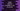# How to check if a number is positive, negative or zero in Kotlin# Introduction :

In this Kotlin programming tutorial, we will learn how to check if a number is positive, negative or zero in Kotlin. We will implement this program with a when block.

The program will ask the user to enter a number. It will read the number, find out if it is negative, positive or zero and print out the result.

## Kotlin program :

``````fun checkInt(i: Int): String {
return when {
i < 0 -> "a negative number"
i > 0 -> "a positive number"
else -> "zero"
}
}

fun main(args: Array) {
val userInput: Int
print("Enter a number : ")
userInput = readLine()?.toInt() ?: 0

println("\$userInput is \${checkInt(userInput)}")
}``````

### Sample Output :

``````Enter a number : -12
-12 is a negative number

Enter a number : 0
0 is zero

Enter a number : 123
123 is a positive number``````

You might also like :